# Section 8.3. Changing the Volume of Sounds

[Page 271 (continued)]

### 8.3. Changing the Volume of Sounds

Earlier, we said that the amplitude of a sound is the main factor in the volume. This means that if we increase the amplitude, we increase the volume. Or if we decrease the amplitude, we decrease the volume.

Don't get confused herechanging the amplitude doesn't reach out and twist up the volume knob on your speakers. If your speaker's volume (or computer's volume) is turned down, the sound will never get very loud. The point is getting the sound itself louder. Have you ever watched a movie on TV where, without changing the volume on the TV, sound becomes so low that you can hardly hear it? (Marlon Brando's dialogue in the movie The Godfather comes to mind.) That's what we're doing here. We can make sounds shout or whisper by tweaking the amplitude.

#### 8.3.1. Increasing Volume

Here's a method that doubles the amplitude of an input sound.

[Page 272]

##### Program 65. Increase an Input Sound's Volume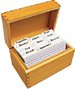`/** * Method to double the volume (amplitude) of the sound */ public void increaseVolume() { SoundSample[] sampleArray = this.getSamples(); SoundSample sample = null; int value = 0; int index = 0; // loop through all the samples in the array while (index < sampleArray.length) { sample = sampleArray[index]; value = sample.getValue(); sample.setValue(value * 2); index++; } }`

Go ahead and type the above into your DrJava definitions pane before the last curly brace in the Sound.java class. Click COMPILE ALL to get DrJava to compile it. Follow along the example below to get a better idea of how this all works.

To use this program, you have to create a sound first and invoke this method on it. Don't forget that you can't type this code in and have it work as-is: Your path names may be different than what is shown here!

`> String f = "c:/intro-prog-java/mediasources/gettysburg10.wav"; > Sound s = new Sound(f); > s.play(); > s.explore(); > s.increaseVolume(); > s.play(); > s.explore();`

In the interactions pane we create a variable f which refers to a String object that holds the name of a file. We create the variable s which refers to a Sound object created from the file using new Sound(f). We ask this Sound object to play using s.play(). We then open an explorer on the sound to see what it looks like graphically using s.explore(). We next increase its volume using s.increaseVolume(). This implicitly passes the Sound object to the method increaseVolume(). So the code this.getSamples() in the method increaseVolume() means to get them from the implicitly passed Sound object (the one referred to by variable s).

[Page 273]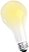## Computer Science Idea: Changing Memory Doesn't Change the File

If you create another Sound object from the same file, will you get the original sound or the sound with volume increased? You will get the original sound. The Sound object s was created by reading the file data into memory. The change to the Sound object was done in memory, but the file wasn't changed. If you want to save your changes write them out to a file using the method soundObj.write(String fileName); where soundObj is the name of the Sound object and fileName is the full path name of the file. So to save the changed Sound object above use s.write("gettyLouder.wav");. This will create a new file with the changed sound in it.

#### 8.3.2. Did that Really Work?

Now, is it really louder, or does it just seem that way? We can check it in several ways. You could always make the sound even louder by evaluating increaseVolume on our sound a few more timeseventually, you'll be totally convinced that the sound is louder. But there are ways to test even more subtle effects.

If you compare graphs of the two sounds using the sound explorer, you will find that the graph of the sound does have greater amplitude after increasing it using our method. Check it out in Figure 8.17.

##### Figure 8.17. Comparing the graphs of the original sound (left) and the louder one (right).Maybe you're unsure that you're really seeing a larger wave in the second picture. You can use a sound explorer to check the individual sample values. You can actually already see that in Figure 8.17see that the first value (index number 0) is 59 in the original sound and 118 in the second sound. You can also check the value at any index using the sound explorer. Just click on a location and the value will be displayed for that location. To check the same location in the second explorer just type in the desired current index and it will show the value at that index. You'll see that the louder sound really does have double the value of the same sample in the original sound (Figure 8.18).

##### Figure 8.18. Comparing specific samples in the original sound (left) and the louder one (right). (This item is displayed on page 274 in the print version)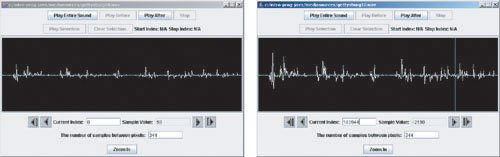Finally, you can always check for yourself from within DrJava. If you've been following along with the example, then the variable s is the now louder sound. f should still be the filename of the original sound. Go ahead and make a new sound object which is the original soundthat is named below as sOrig (for sound original). Check any sample that you wantit's always true that the louder sound has twice the value than the original sound.

 What? You haven't? You should! It'll make much more sense if you try it yourself!

[Page 274]

`> System.out.println(s);  Sound file: c:/intro-prog-java/mediasources/gettysburg10-louder.wav number of samples: 220568 > System.out.println(f); c:/intro-prog-java/mediasources/gettysburg10.wav > Sound sOrig = new Sound(f); > System.out.println(s.getSampleValueAt(0)); 118 > System.out.println(sOrig.getSampleValueAt(0)); 59 > System.out.println(s.getSampleValueAt(1)); 78 > System.out.println(sOrig.getSampleValueAt(1)); 39 > System.out.println(s.getSampleValueAt(999)); -80 > System.out.println(sOrig.getSampleValueAt(999)); -40`

You can see from the last value that even negative values become more negative. That's what's meant by "increasing the amplitude." The amplitude of the wave goes in both directions. We have to make the wave larger in both the positive and negative dimensions.

It's important to do what you just read in this chapter: Doubt your programs. Did that really do what we wanted it to do? The way you check is by testing. That's what this section is about. You just saw several ways to test:

• By looking at the result overall (like with the graphs created by the explorer),

• By checking pieces of the results (like with the explorer or MediaTools), and

• [Page 275]
• By writing additional code statements that check the results of the original program.

##### Figuring out how it worked

Let's walk through the code, slowly, and consider how this program worked.

`/**  * Method to double the volume (amplitude) of the sound  */ public void increaseVolume() {   SoundSample[] sampleArray = this.getSamples();   SoundSample sample = null;   int value = 0;   int index = 0;   // loop through all the samples in the array   while (index < sampleArray.length)   {     sample = sampleArray[index];     value = sample.getValue();     sample.setValue(value * 2);     index++;   } }`

Recall our picture of the samples in a sound array.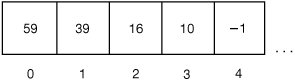This is what sound.getSamples() would return: An array of SoundSample objects. The while loop allows us to walk through each sample, one at a time. The name (variable) sample will refer to each SoundSample object in turn.

The variable index starts out with a value of 0. This is less than the length of the array sampleArray so the body of the loop is executed. The variable sample is changed to refer to the first SoundSample object (the one at index 0).The variable value will take on the value of 59 when value=sample.get Value() is executed. The value stored at that SoundSample object will be set to value times 2 (59 * 2 = 118).

[Page 276]The value in variable index will be incremented by 1 (0 + 1 = 1). That's the end of the first pass through the body of the while loop. The loop will then start over. The test that index is less than the length of the array of samples will happen again. Since it is still less the body of the loop will be executed (statements inside the open and close curly braces). The variable sample will be changed to refer to the second item in the array (the one at index 1).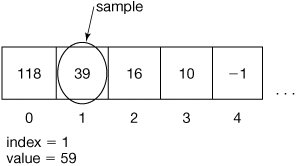Again, the variable value is set to the value of the SoundSample object. The value of the SoundSample object is set to twice the amount held in the variable value.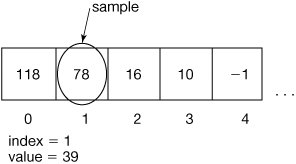This is what it will look like after five times through the loop.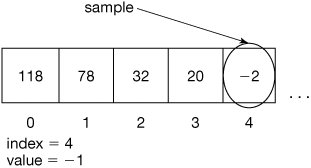[Page 277]

But really, the while loop keeps going through all the samplestens of thousands of them! Thank goodness it's the computer executing this program!

What you have just read in this section is called tracing the program. We slowly went through how each step in the program was executed. We drew pictures to describe the data in the program. We used numbers, arrows, equations, and even plain English to explain what was going on in the program. This is the single most important technique in programming. It's part of debugging. Your program will not always work. Absolutely, guaranteed, without a shadow of a doubtyou will write code that does not do what you want. But the computer will do SOMETHING. How do you figure out what it is doing? You debug, and the most significant way to do that is by tracing the program.

#### 8.3.3. Decreasing Volume

Decreasing volume, then, is the reverse of the previous process.

##### Program 66. Decrease an Input Sound's Volume`/** * Method to halve the volume (amplitude) of the sound. */ public void decreaseVolume() { SoundSample[] sampleArray = this.getSamples(); SoundSample sample = null; int value = 0; int index = 0; // loop through all the samples in the array while (index < sampleArray.length) { sample = sampleArray[index]; value = sample.getValue(); sample.setValue((int) (value * 0.5)); index++; } }`

• Our method is called on a Sound object. The Sound object is implicitly passed to the method and is accessed using the keyword this. You can leave off the this on this.getSamples() since it is understood to be invoked on the current object.

• The variable sample will refer to a different SoundSample object each time through the loop.

• Each time sample refers to a new SoundSample object, we will get the value of that SoundSample object. We put that in the variable value.

• [Page 278]
• We then set the value held by the SoundSample object to 50% of its current value, by multiplying value by 0.5, and setting the sample value to that. However, because the value is an integer and the result of a computation with a floating point value (0.5) is a floating point number we must cast to integer using (int) (value * 0.5) to let the compiler know we realize that we will be throwing away the fractional part.

We can use it like this.

`> String f = FileChooser.pickAFile(); > System.out.println(f); C:\intro-prog-java\mediasources\gettysburg10-louder.wav > Sound sound1 = new Sound(f); > System.out.println(sound1); Sound file: C:\intro-prog-java\mediasources\gettysburg10-louder.wav number of samples: 220568 > sound1.play(); > sound1.decreaseVolume(); > sound1.play();`

We can even do it again, and lower the volume even further.

`> sound1.decreaseVolume(); > sound1.play();`

#### 8.3.4. Using a for Loop

Have you ever forgotten to declare the variable index? If you did the method wouldn't compile. Did you ever forget to increment the variable index? If you did the loop would never end until you hit RESET. Because of these problems, programmers typically use a for loop instead of a while loop when they want to execute a block of commands a set number of times. A for loop is equivalent to a while loop (means the same thing to the computer). The for loop is just less error prone for a programmer (though it can be harder for a beginner to understand). We introduced for loops in Section 4.3.7.

A for loop looks like this: for (initialization; test; change). The initialization area lets you declare and initialize variables for use in the loop, the test is where you test if the loop should continue, and the change area is where you change the value of counters or indices used in the loop. For example, see the following new version of the method decreaseVolume which has been modified to use a for loop instead of a while loop.

##### Program 67. Decrease an Input Sound's Volume Using a for Loop (This item is displayed on pages 278 - 279 in the print version)`/** * Method to halve the volume (amplitude) of the sound. */ public void decreaseVolume2() { [Page 279] SoundSample[] sampleArray = this.getSamples(); SoundSample sample = null; int value = 0; // int index = 0; // loop through all the samples in the array // while (index < sampleArray.length) for (int index = 0; index < sampleArray.length; index++) { sample = sampleArray[index]; value = sample.getValue(); sample.setValue((int) (value * 0.5)); // index++; } }`

We have used the to-end-of-line comment '//' to comment out some lines of code to show the difference between the while and for loops. Notice that what is different is that we don't declare and initialize the index before the loop, it is done in the initialization part of the for statement. We also don't increment the index as the last statement in the loop. This is moved to the change area in the for statement. So we have replaced three lines of code with one and made it more likely that we will remember to declare variables for use in the loop and change them. However, what really happens during execution is the same thing as what happened during the while loop. The declarations and initializations done in the initialization part of the for loop will actually take place before the first test. The change of the loop variables will actually take place after each execution of the loop body and before the next test.

#### 8.3.5. Making Sense of Methods

The lessons that we learned when writing picture methods (from Section 4.3.5) apply to sound methods as well. We want to write methods that do one and only one thing. We want to write methods that can be reused.

We can write methods that take an input value. For example, here's a program to changeVolume. It accepts a factor that is multiplied by each sample value. This method can be used to increase or decrease the amplitude (and thus, the volume).

##### Program 68. Change a Sound's Volume by a Given Factor (This item is displayed on pages 279 - 280 in the print version)`/** * Method to change the volume (amplitude) of the sound * by multiplying the current values in the sound by * the passed factor. * @param factor the factor to multiply by */ public void changeVolume(double factor) { SoundSample[] sampleArray = this.getSamples(); [Page 280] SoundSample sample = null; int value = 0; // loop through all the samples in the array for (int i = 0; i < sampleArray.length; i++) { sample = sampleArray[i]; value = sample.getValue(); sample.setValue((int) (value * factor)); } }`

This program is clearly more flexible than increaseVolume(). Does that make it better? Certainly it is for some purposes (e.g., if you were writing software to do general audio processing), but for other purposes, having separate and clearly named methods for increasing and decreasing volume may be better. Of course, you could modify increaseVolume() and decreaseVolume() to call changeVolume() with the appropriate factor. Remember that software is written for humanswrite software that is understandable for the people who will be reading and using your software.

We are reusing the name sample a lot. We have used it in several methods in the Sound class. That's okay. Names can have different meanings depending on their context. Variables declared in a method have meaning only inside that method. Methods can even use the same variable names as other methods. You can even use the same variable names that you use in your methods in the interactions pane. This is a different context. If you create a variable in a method context (like value in Program 68 above), then that variable won't exist when you get back out to the interactions pane. We can return values from a method context back out to the interactions pane (or a calling method) by using return, which we'll talk more about later.Introduction to Computing & Programming Algebra in Java(c) A Multimedia Approach
ISBN: N/A
EAN: N/A
Year: 2007
Pages: 191